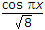# Electronics and Communication Engineering - Microwave Communication

### Exercise :: Microwave Communication - Section 5

26.

The rotation of direction of E field of a linearly polarized wave passing through a magnetised ferric medium is called

 A. Lorentz rotation B. Faraday rotation C. Steinmitz rotation D. Fleming's rotation

Answer: Option B

Explanation:

No answer description available for this question. Let us discuss.

27.

If air pressure in a rectangular waveguide is increased the maximum power handling capacity

 A. is decreased B. is increased C. remains the same D. may increase or decrease depending on pressure

Answer: Option B

Explanation:

No answer description available for this question. Let us discuss.

28.

A directional coupler is used to determine

 A. rv B. rv and VSWR C. L and C D. wave velocity

Answer: Option B

Explanation:

No answer description available for this question. Let us discuss.

29.

A square waveguide carries TE11 mode whose axial magnetic field is given by Hz = H0,where the guide dimensions are in cms. The cut off frequency of the mode is

 A. 5 GHz B. 7.5 GHz C. 6.5 GHz D. 8 GHz

Answer: Option B

Explanation:

No answer description available for this question. Let us discuss.

30.

For air filled circular waveguides

 A. the attenuation due to imperfect conducting walls is negligible B. the attenuation due to imperfect dielectric is negligible C. both the attenuation i.e. due to imperfect conducting walls and due to imperfect dielectric are significant D. the total attenuation is almost zero

Answer: Option B

Explanation:

No answer description available for this question. Let us discuss.

#### Current Affairs 2021

Interview Questions and Answers